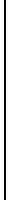# Statistics of identities of the classical vector analysis in R3

### May, 2001

The following table contains statistics about identities which exist between so-called standard terms of the classical vector analysis in R3. Standard terms formed from k vectors and 1 test vector x are for instance

k + 1 = 4 k + 1 = 5
<a,b><c,x>
<a,c><b,x>
<a,x><b,c>


Examples of identities between standard terms are for instance

<a,d> b × c + <b,d> c × a + <c,d> a × b  =  <a,b,c> d          (an identity which does not contain a test vector x)

and=   0 .

More details can be found on the page of PERMS notebooks where I present the text of a talk (given at SLC46, Lyon, 2001) and the Mathematica notebooks of the calculation of the following table.

 k + 1 l blocks # ideals dim terms # ident # summands 4 (4) (2,2) (4) (2,2) 1 1 1 2 3 0 --- 5 (3,1,1) (3,1,1) 1 6 10 4 4 6 (6) (4,2) (2,2,2) (6) (4,2) (2,2,2) 1 1 1 1 9 5 15 0 --- 7 (5,1,1) (3,3,1) (5,1,1) (3,3,1) 1 1 15 21 105 69 4, 10, 12, 14, 16, 18 8 (8) (6,2) (4,4) (4,2,2) (8) (6,2) (4,4) (4,2,2) 1 1 1 1 1 20 14 56 105 14 24, 36, 40, 44, 50, 52 9 (7,1,1) (5,3,1) (3,3,3) (7,1,1) (5,3,1) (3,3,3) 1 1 1 28 162 42 1260 1028 ???

 k number of vectors which are used to form standard expressions (k vectors + 1 test vector x) l partitions which are grouping partitions for a given k blocks partitions which denote minimal two-sided ideals of the group ring in which the characterizing spaces W have non-trivial projections # ideals number of minimal right ideals which form the projection of W in the two-sided ideal denoted by 'blocks' dim dimension of the minimal right ideals from the column '# ideals' terms number of standard terms for a given k # ident number of linearly independent identities for a given k # summands number of summands which I found in a set of linearly independent identities